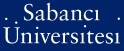# Modeling and estimation of the dynamics of planar algebraic curves via riccati equations

Ünel, Mustafa and Ghosh, Bijoy K. and Yazıcıoğlu, Ahmet Y. (2010) Modeling and estimation of the dynamics of planar algebraic curves via riccati equations. Journal of Mathematical Imaging and Vision, 38 (2). pp. 139-158. ISSN 0924-9907 (Print) 1573-7683 (Online)

Full text not available from this repository.

Official URL: http://dx.doi.org/10.1007/s10851-010-0209-3

## Abstract

Motivated by problems in vision and robotics, in this paper we are interested in describing the dynamics of planar algebraic curves in rigid and affine motion. A new method is presented for modeling the dynamics of such curves in terms of Riccati equations. It is shown that rigid or affine motion of an algebraic curve can be described using the dynamics of line factors obtained from a unique decomposition of the curve, and each individual line dynamics can be described by a Riccati equation. An estimation algorithm is proposed to estimate rigid or affine motion using line parameters. Importance of data normalization is also investigated in the context of motion estimation. Experiments with simulated data and real images demonstrate that the proposed method can provide satisfactory motion estimation results from perturbed data.

Item Type: Article Motion estimation; Algebraic curves; Affine motion; Riccati equations; Data normalization Q Science > QA Mathematics > QA075 Electronic computers. Computer science 14303 Mustafa Ünel 31 Aug 2010 16:39 25 Jul 2019 12:38

Repository Staff Only: item control page